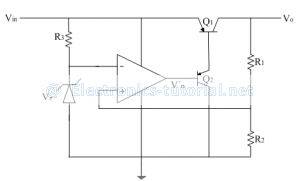Home > analog integrated circuits > voltage regulator > low drop out voltage regulators

# Low drop-out Voltage regulators

The dropout voltage is the minimum voltage that must exist between input and output for proper functioning of the regulator.
For IC 7805, the dropout voltage is VDO=2.5V
∴Vin=5+2.5=7.5V
In car batteries the input can drop to a very low value in cold conditions; but its efficient operation is very much essential. In such cases low dropout voltage regulators are used.
The following figure shows low dropout regulator using op-amp.The voltage at inverting terminal of op-amp is the zener voltage Vz. Due to virtual ground concept the non-inverting terminal is also appeared to be at same potential i.e. V+. Thus the voltage Vz is given as
V^+=Vz=[Vo/(R1+R2 )] R2
∴Vo=[(R1+R2)/R2 ] V^+
∴Vo=[1+R1/R2 ] V^+